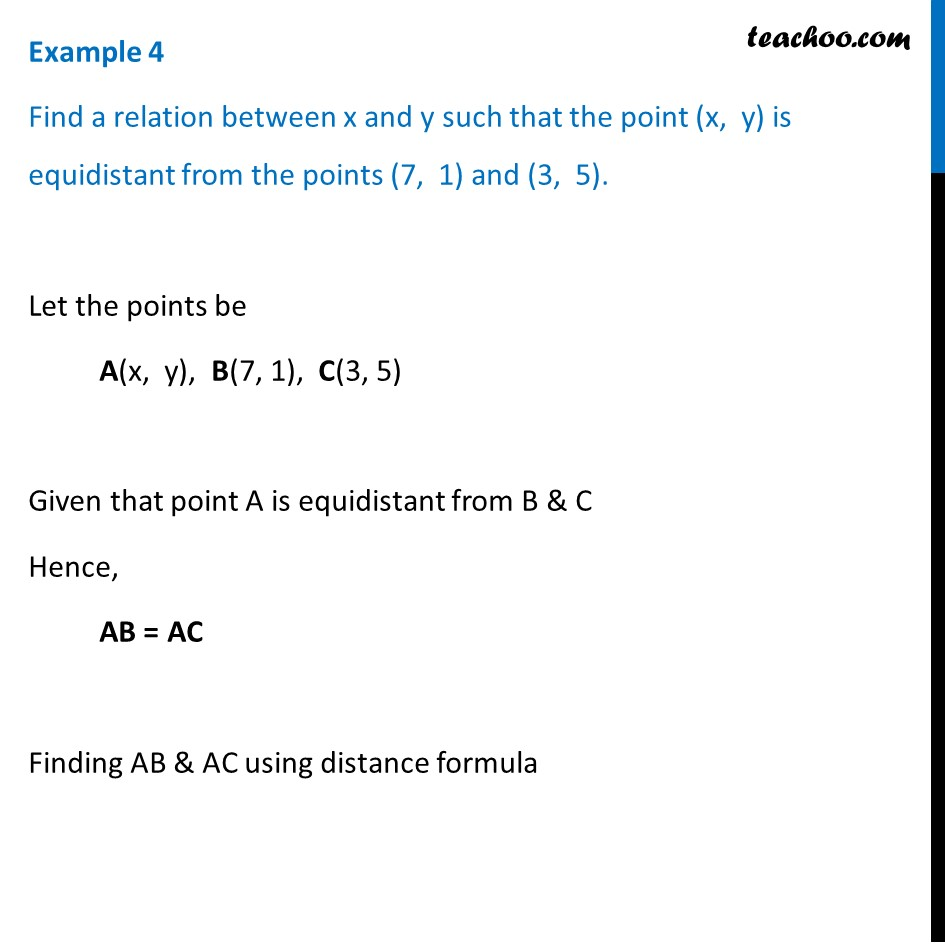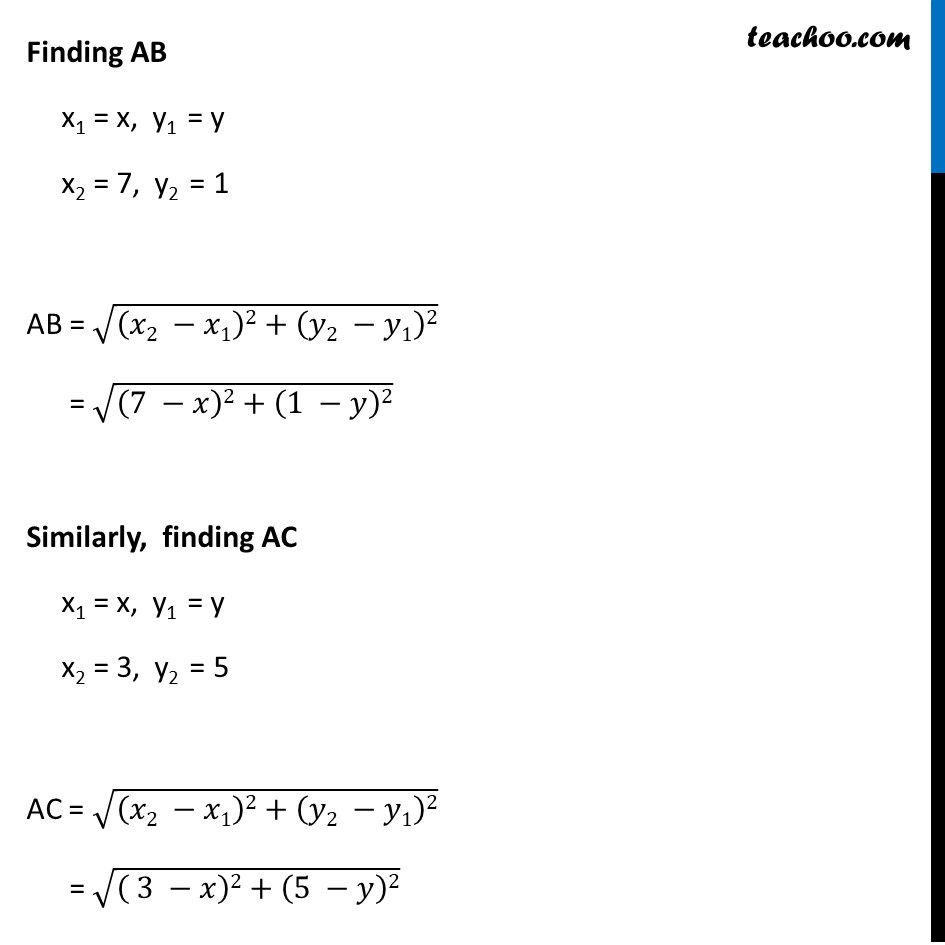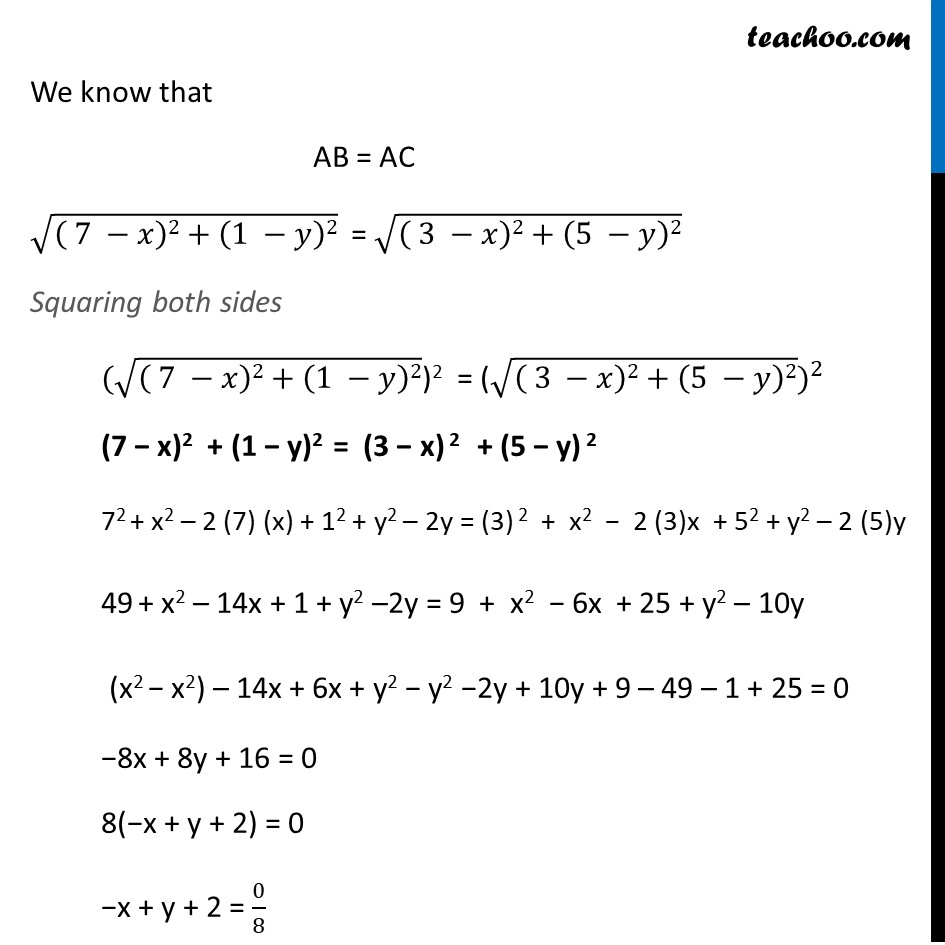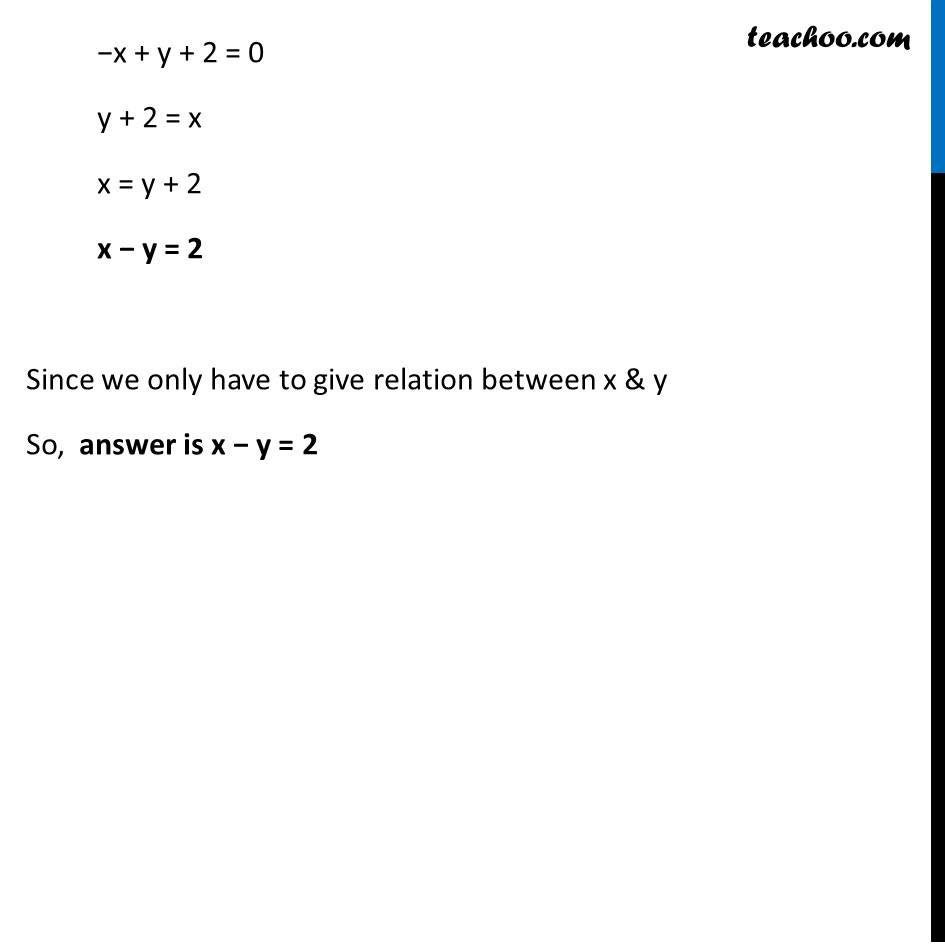Examples

Chapter 7 Class 10 Coordinate Geometry
Serial order wiseLearn in your speed, with individual attention - Teachoo Maths 1-on-1 Class

### Transcript

Example 4 Find a relation between x and y such that the point (x, y) is equidistant from the points (7, 1) and (3, 5). Let the points be A(x, y), B(7, 1), C(3, 5) Given that point A is equidistant from B & C Hence, AB = AC Finding AB & AC using distance formula Finding AB x1 = x, y1 = y x2 = 7, y2 = 1 AB = √((𝑥2 −𝑥1)2+(𝑦2 −𝑦1)2) = √((7 −𝑥)2+(1 −𝑦)2) Similarly, finding AC x1 = x, y1 = y x2 = 3, y2 = 5 AC = √((𝑥2 −𝑥1)2+(𝑦2 −𝑦1)2) = √(( 3 −𝑥)2+(5 −𝑦)2) We know that AB = AC √(( 7 −𝑥)2+(1 −𝑦)2) = √(( 3 −𝑥)2+(5 −𝑦)2) Squaring both sides 〖(√(( 7 −𝑥)2+(1 −𝑦)2) ")2 = (" √(( 3 −𝑥)2+(5 −𝑦)2))〗^2 (7 − x)2 + (1 − y)2 = (3 − x) 2 + (5 − y) 2 72 + x2 – 2 (7) (x) + 12 + y2 – 2y = (3) 2 + x2 − 2 (3)x + 52 + y2 – 2 (5)y 49 + x2 – 14x + 1 + y2 –2y = 9 + x2 − 6x + 25 + y2 – 10y (x2 − x2) – 14x + 6x + y2 − y2 −2y + 10y + 9 – 49 – 1 + 25 = 0 −8x + 8y + 16 = 0 8(−x + y + 2) = 0 −x + y + 2 = 0/8 −x + y + 2 = 0 y + 2 = x x = y + 2 x − y = 2 Since we only have to give relation between x & y So, answer is x − y = 2